Area of parallelogram

Chapter 9 Class 7 Perimeter and Area
Concept wise

A parallelogram has opposites sides equal & parallel

So, in parallelogram ABCD

AB = CD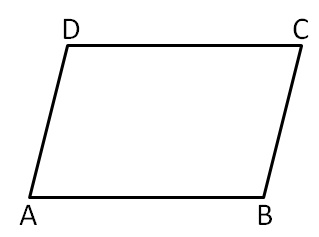Area of parallelogram is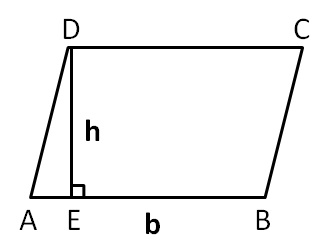Area of parallelogram = Base × Height

= b × h

Here,

Base = AB = b

Height = DE = h

Here,

Base is any side of parallelogram

& Height is perpendicular from opposite vertex to the base

So,

Area of parallelogram can be written as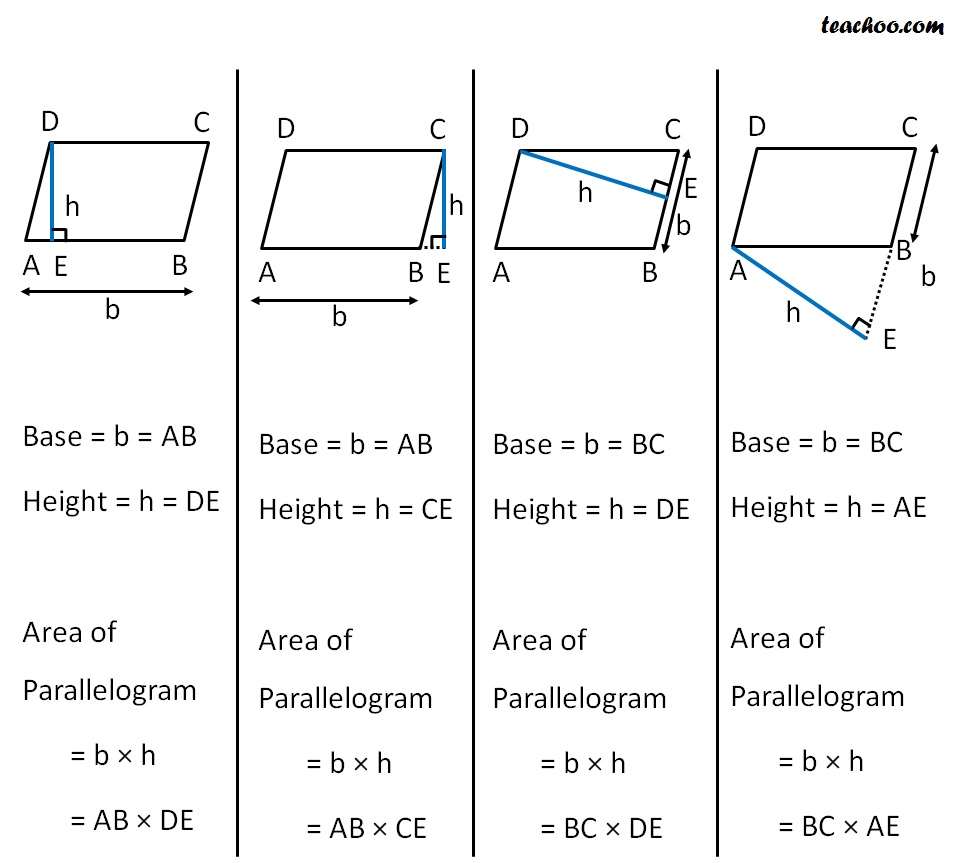Let’s take some examples,

#### Find area of parallelogram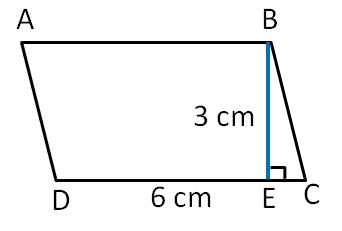Base = b

= CD

= 6 cm

Height = h

= BE

= 3 cm

Area of parallelogram = b × h

= 6 × 3

= 18 cm 2

#### Find area of parallelogram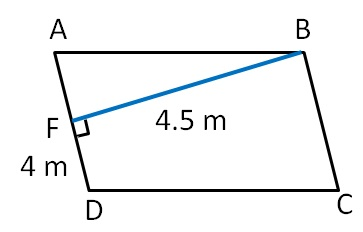Base = b

= 4 m

Height = h

= BF

= 4.5 m

Area of parallelogram = b × h

= 4 × 4.5

= 18 m 2

#### Find area of parallelogram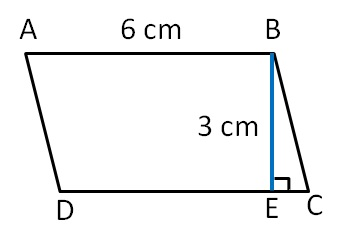Base = b

= DC

= AB                           (Opposite sides of parallelogram are equal)

= 6 cm

Height = h

= BF

= 3 cm

Area of parallelogram = b × h

= 6 × 3

= 18 cm 2

#### Find area of parallelogram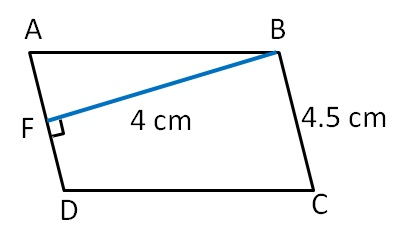Base = b

= BC

= 4.5 cm

Height = h

= BF

= 4 cm

Area of parallelogram = b × h

= 4.5 × 4

= 18 cm 2

Learn in your speed, with individual attention - Teachoo Maths 1-on-1 Class

### Transcript

Base = b = AB Height = h = DE Area of Parallelogram = b × h = AB × DE Base = b = AB Height = h = CE Area of Parallelogram = b × h = AB × CE Base = b = BC Height = h = DE Area of Parallelogram = b × h = BC × DE Base = b = BC Height = h = AE Area of Parallelogram = b × h = BC × AE Let’s take some examples,